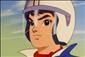# Arrays in Java: Non-Rectangular Arrays (Part-5)

So far we have seen the declaration arrays and use of rectangular arrays. Java allows us to create non-rectangular arrays. A nonrectangular array is an array in which each row of the array may have a different number of columns. The memory layout for a nonrectangular array A :

##### Array A
 5 2 18 -6 -3 -7 8 9 1 -4 3 7 -2 -8

·    Array A is a nonrectangular array having four rows.

·     When allocated in memory, the variable A will refer to a contiguous memory allocation of four cells.

·     Each cell holds a reference to another single-dimensional array.

·     In our case, the first cell holds a reference to an array having five elements.

·     The second row holds a reference to an array having two elements.

·      The third row holds a reference to an array having three rows.

·      And finally the fourth cell holds a reference to an array having four rows.

##### Runtime Initialization

Consider a two-dimensional array whose first dimension is, let’s say, 5. Therefore, the array will contain five rows. We may now declare different column sizes for each row. For example, the first row may contain four columns; the second row may contain three columns, and so on. Such a declaration is shown here:

```
int[ ][ ] jaggedArray = new int[ ];
jaggedArray = new int;
jaggedArray = new int;
jaggedArray = new int;
jaggedArray = new int;
jaggedArray = new int;```

The jaggedArray is a two-dimensional array, as declared in the first statement. During the declaration, we initialize the first dimension of the array to 5, indicating that the array would contain five rows. Here, we do not specify the value for the second dimension.

On the next line, we initialize the first row of the jaggedArray. Each row consists of a certain number of cells of type int. The number of cells in each row is the column length of the array. We initialize this to 4. Thus, the first row (that is, the row with ID 0) would contain four columns.

For the second row (row ID 1), we declare the column size 3. The second row therefore contains three columns. Likewise, for third row, we set a column size of 5; for the fourth row the column size is 2, and for the fifth row the column size is 4.

To access the elements of this array, we would use the syntax jaggedArray[i][j], as in the case of the earlier examples. You will have to ensure that i, j values are within the range of the array declaration. For example, jaggedArray is valid, whereas jaggedArray is invalid because the second row contains only three columns.

This type of nonrectangular array is also called a “jagged” or “ragged” array. Sometimes, it is also called an “array of arrays.” Note that like rectangular arrays (that is, arrays having the same number of columns in each row), each element of the nonrectangular array must be of the same data type.

##### Initialization Using Array Literals

The same way we initialize single-dimensional and other rectangular arrays using array literals, we can initialize a non-rectangular array. Consider the following declaration:

int[ ][ ] myArray = {{3, 4, 5}, {1, 2} };

Here, myArray is a two-dimensional array of integers. The first row of the array consists of three elements, whereas the second row consists of only two elements. Thus, this is a nonrectangular array. While accessing the elements of this array, we will have to take care to ensure that both indices lie within the bounds of the array for each row.

Last updated:3/28/2020 2:47:30 AM#### David Miller